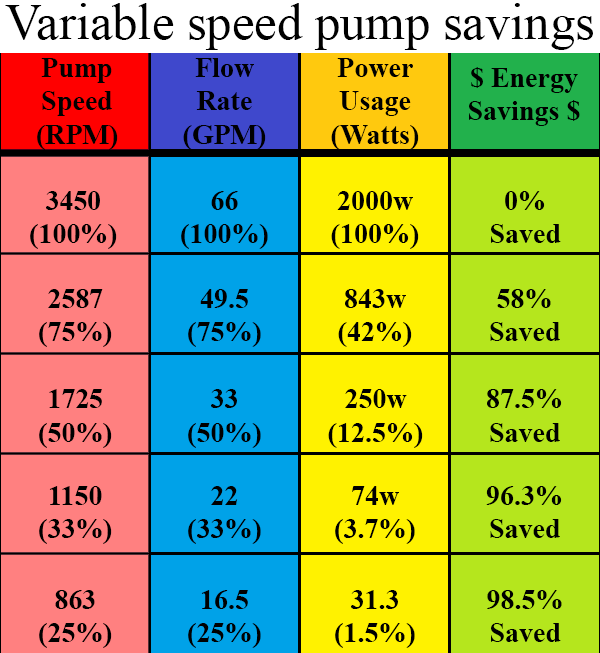Search
•Nick Young

# Variable speed pumps help save this summer

With summer right around the corner, its safe to assume that your power bill will go up quite a bit thanks to the A/C and pool pump alone. While its pretty obvious that the air conditioning is the highest energy user in the home, most people don't realize their pool pump is the second highest. Central air uses about 15,000w per hour (15kWh), a single speed pool pump uses about 3,000w per hour (3kWh) and as comparison, a refrigerator uses about 1,000w per hour (1kWh) and a computer uses 400w (0.4kWh).

Energy cost per hour varies between providers and between tiers, but in southern Orange County Ca. Southern California Edison charges \$0.17 per kWh in tier 1, \$0.25 per kWh in tier 2, and \$0.35 per kWh in tier 3. This means depending on which tier you are in, running your pool pump for 8 hours per day can cost \$4.08 per day (\$0.17 x 3kWh x 8hr) up to \$8.40 per day (\$0.35 x 3kWh x 8hr). This means you could be paying \$1,489.20 to \$3,066 a year JUST TO RUN THE PUMP.

Variable speed pumps change the game, in a big savings kind of way. The difference between a variable speed pump and a single speed pump sounds pretty self explanatory, and it is. A single speed pump runs at full speed, all the time. A variable speed pump can run at any speed between off and full. This is beneficial because the scale of speed to energy used is not linear.

The physics of pumps (Affinity Law) can be confusing but essentially states that flow rate ( F ) is equal to pump speed ( S ) or (Flow = Speed) , pressure ( P ) is the square of the pump speed (P = SxS), and energy used (E) is the cube of the pump speed (E = SxSxS). To put it simply, if you run a pump at half speed (S = .5 or 50%) you will get half the flow (F=.5 or 50%) one quarter of the pressure (P = .5 x .5 =.25 or 25%) and use one eighth the energy (E = .5 x .5 x .5 = .125 or 12.5%)

So lets make an example, lets use the numbers from before and say a 1HP single speed pump uses 3kWh and runs for 8 hours a day. So, 100% speed = 100% flow = 100% pressure = \$1,489 per year (tier 1 rate). Lets compare it to a variable speed running at half the speed. So, 50% speed = 50% flow = 25% pressure = \$186.125 per year (tier 1 rate). That is a huge savings when running the pump at just half the speed. Now do keep in mind, the goal of the pump is to filter the water so we want to run the pump twice as long when we run it at half the speed.

The concept of affinity law can be a difficult one to wrap your head around, especially without digging into physics too deeply, but the important part is remembering that reducing the speed by half, does not mean you use half the energy, its half times half times half. You could even take the concept a step further and run the pump at 1/3 (33% or .33) the speed, but you would use .33 x .33 x .33 = .035 or 3.5% the energy, or 3.5%. Just remember you need to get the same filtration, so you would triple the run time so 10.5% or 89.5% energy savings.

While the summer usually means higher energy bills, and the price of variable speed pumps may look intimidating, with energy company rebates and the ability to use a fraction of the energy while keeping the pool just as clean, you will make your money back in no time. And as a last little tip for you, affinity law applies to fans too, meaning, you can reduce the speed of your fan to use a fraction of the energy and keep the pocket book sting out of summer.